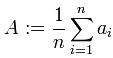### Introduction

In mathematics and statistics, the arithmetic mean, or simply the mean or average when the context is clear, is the central tendency of a collection of numbers taken as the sum of the numbers divided by the size of the collection.

### Definition

Suppose we have sample space {a1 , ... , an}. Then the arithmetic mean A is defined via the equation:If the list is a statistical population, then the mean of that population is called a population mean. If the list is a statistical sample, we call the resulting statistic a sample mean.

The arithmetic mean of a variable is often denoted by a bar, for example(read "x bar") would be the mean of the sample space X.

### References & Resources

• http://en.wikipedia.org/wiki/Arithmetic_mean